Subset, Power Set & Universal Set

# Subset, Power Set & Universal Set - Notes | Study Mathematics (Maths) Class 11 - Commerce

 Table of contentsSubsetSupersetPower SetUniversal SetPractice Questions1 Crore+ students have signed up on EduRev. Have you?

Subset
A set X is a subset of set Y if every element of X is also an element of Y.
In symbol, we write x ⊆ y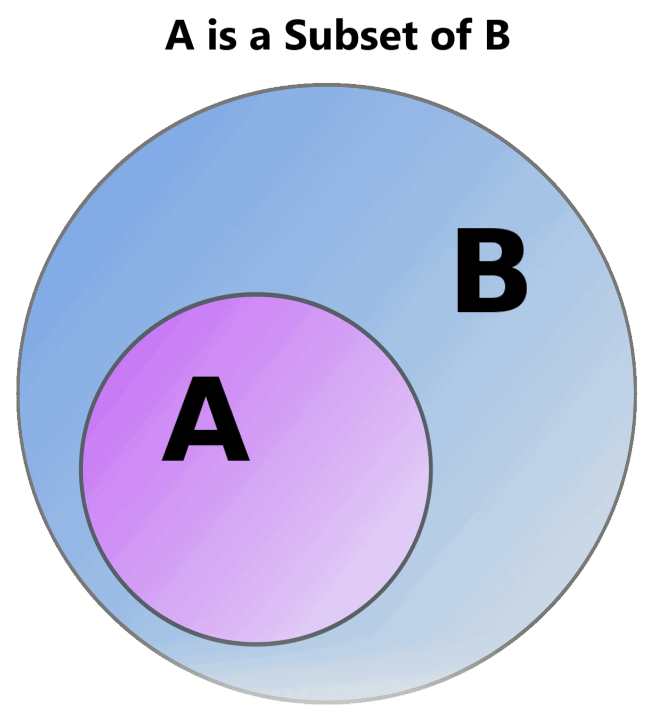Reading Notation: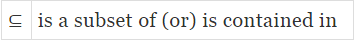Read ⊆ as "X is a subset of Y" or "X is contained in Y"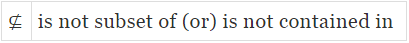Read ⊈ as "X is a not subset of Y" or "X is not contained in Y".
The formula for calculating the number of subsets = 2n, where n = number of elements in the set.

Note:

• If A and B are any two non-empty sets such that A ⊆ B Let x be any element such that x ∈ A ⇒ x ∈ B
• Every set is a subset of itself that means A ⊆ A
• An empty set i.e ∅ is a subset of every set

Representation of the Subsets of Real Numbers

Intervals as subsets of R:

1. Closed interval: Let a and b are two real numbers such that a < b. Then the set of all real numbers x such that a ≤ 𝑥 ≤ b is called closed interval and denoted by [a, b].
Thus [a, b] = {x : x ∈ 𝑅, a ≤ 𝑥 ≤ b}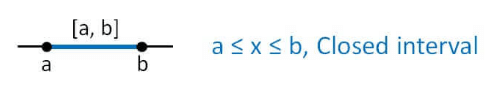2. Open interval: similarly open intervals are denoted by (a, b) where
(a, b) = {x : x ∈ 𝑅, a < 𝑥 < b }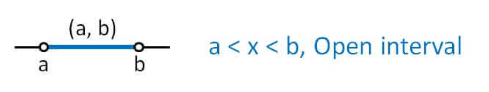3. Semi-open or semi-closed interval: here these intervals are denoted by (a, b] or [a, b)
(a, b] ={x : x∈ 𝑅, a < 𝑥 ≤ b} or [a, b) = {x : x ∈ 𝑅, a ≤ 𝑥 < b}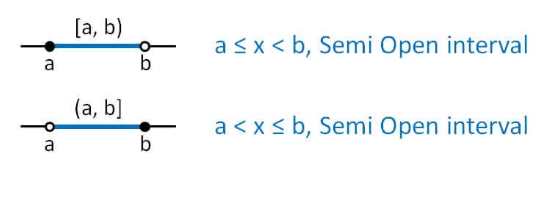Example.1 Which of the following are correct statements?
A = {1, 2, 3, 4, 5, 6}
(a) {2, 3} ⊂ A
(b) {1, 2, 3, 4, 5,6, 7} ⊃ A
(c) 8 ⊂ A
(d) {3, 5, 1, 7} ⊃ A
(e) {1} ⊂ A
(f) {1, 2, 3, 4} ⊂ A
(g) { } ⊃ A
(h) ϕ ⊂ A

Ans. (a), (b), (e), (f), (h)

Types of Subsets

Subsets are classified as:

1. Proper Subset
2. Improper Subsets

A proper subset is one that contains a few elements of the original set whereas an improper subset, contains every element of the original set along with the null set.
Example:
If set A = {2, 4, 6}, then,

Number of subsets: {2}, {4}, {6}, {2,4}, {4,6}, {2,6}, {2,4,6} and Φ or {}.

Proper Subsets: {}, {2}, {4}, {6}, {2,4}, {4,6}, {2,6}

Improper Subset: {2,4,6}

1. Proper Subsets

• Set A is considered to be a proper subset of Set B if Set B contains at least one element that is not present in Set A.
• Example: If set A has elements as {12, 24} and set B has elements as {12, 24, 36}, then set A is the proper subset of B because 36 is not present in the set A.
• A proper subset is denoted by ⊂ and is read as ‘is a proper subset of’. Using this symbol, we can express a proper subset for set A and set B as:
A ⊂ B
• If we have to pick n number of elements from a set containing N number of elements, it can be done in NCn number of ways.
• Therefore, the number of possible subsets containing n number of elements from a set containing N number of elements is equal to NCn.

How many subsets and proper subsets does a set have?

• If a set has “n” elements, then the number of subset of the given set is 2n and the number of proper subsets of the given subset is given by 2- 1.
• Example: If set A has the elements, A = {a, b}, then the proper subset of the given subset are { }, {a}, and {b}. Here, the number of elements in the set is 2.
We know that the formula to calculate the number of proper subsets is 2n – 1.
= 2– 1 = 4 – 1 = 3
Thus, the number of proper subset for the given set is 3 ({ }, {a}, {b}).

2. Improper Subset

• A subset that contains all the elements of the original set is called an improper subset. It is denoted by ⊆.
Example: Set P ={2,4,6} Then, the subsets of P are;
{}, {2}, {4}, {6}, {2,4}, {4,6}, {2,6} and {2,4,6}.
Where, {}, {2}, {4}, {6}, {2,4}, {4,6}, {2,6} are the proper subsets and {2,4,6} is the improper subsets. Therefore, we can write {2,4,6} ⊆ P.

Note: The empty set is an improper subset of itself (since it is equal to itself) but it is a proper subset of any other set.

Question for Subset, Power Set & Universal Set
Try yourself:Let A = {1, 2, 3, 4, 5, 6, 7, 8, 9, 10}. Then the number of subsets of A containing two or three elements is

Superset

• In set theory, set A is considered as the superset of B, if all the elements of set B are the elements of set A.
• Example: If set A = {1, 2, 3, 4} and set B = {1, 3, 4}, we can say that set A is the superset of B. As the elements of B [(i.e.,)1, 3, 4] are in set A. We can also say that B is not a superset of A.
• A set X is said to be a proper subset of set Y if X ⊆ Y and X ≠ Y.
In symbol, we write X ⊂ Y
• Here, Y is called superset of X
Y ⊇ X i.e. Y is the superset of X or Y contains X.

Power Set

• The set of all subsets of A is said to be the power set of the set A.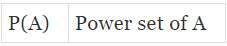The power set of A is denoted by P(A)
• Example: Z = {1, 3, 5}
Here, the number of the elements of Z is 3.
The subsets of Z are { }, {1}, {3}, {5}, {1, 3}, {3, 5}, {1, 5}, {1, 3, 5}
The power set of Z is the set of all the subsets of Z. We will list all the subsets then enclose them in the curly braces “{ }”
P(Z) = { { }, {1}, {3}, {5}, {1, 3}, {3, 5}, {1, 5}, {1, 3, 5} }

Hence n(P(z)) = 2𝑛, where n is the number of elements in set Z.

Question for Subset, Power Set & Universal Set
Try yourself: If set X = {2,3,5,7}, then n[P(X)] is ______. (P denotes Power Set)

Universal Set

• The universal set is the super set of all the elements under consideration. If we are talking about no. of people in India, China and Japan then the universal set will be the set of all the people of these three countries.
• Example: N = {1, 2, 3, 4, 5,…}, A = {1, 3, 5, 7,…} & B = {2, 4, 6, 8,…}
Here in Set A and B we are talking about the odd numbers and even numbers so N i.e. the set of natural numbers will be the universal set of A and B. As it includes all the elements of A and B.

Practice Questions

Q.1. How many elements are there in power set if
(a) A = {ϕ}
(b) B = {a, b}
(c) C = {l, m, n}
(d) D = {4, 9}

Ans.
(a) Here n(A) = 0 ,so n(P(A)) = 20 = 1
(b) 4
(c) 8
(d) 4

Q.2. Find the number of proper subsets of the following.
(a) P = {x : x ∈ N, x < 5}
(b) Q = {x : x is an even prime number}
(c) R = {x : x ∈ W, x < 2}
(d) T = { }
(e) X = {0}
(f) Y = {x : x is prime, 2 < x < 10}

Ans.
(a) 15
(b) 1
(c) 3
(d) 0
(e) 1
(f) 7

Q.3. Write down all the subsets of
(a) {8}
(b) {p, q}
(c) {1, 3, 5}
(d) ϕ
Ans.

(a) ∅, {8}
(b) ∅, {p}, {q}, {p, q}
(c) ∅, {1}, {3}, {5}, {1, 3}, {1, 5}, {3, 5}, {1, 3, 5}
(d)

Q.4. What universal sets would you propose for the following?
(a) The set of all squares
(b) The set of all even numbers
(c) The set of all isosceles triangles
(d) The set of all negative integers
(e) The set of all prime numbers
(f) The set of all obtuse angled triangles
Ans.

(b) N
(c) {triangles}
(d) I
(e) N
(f) {triangles}

Q.5. Fill in the blank spaces using the symbols ⊂ or ⊄.
(a) {1, 2, 3} ______ {1, 3, 5}
(b) ϕ ______ {4, 7, 9}
(c) {x : x is rectangle in a plane} ______ {x : x is a quadrilateral in a plane}
(d) {x : x is an odd natural number} ______{x : x is an integer}
(e) {x : x is a prime number} ______ {x: x is a composite number}
(f) {5, 10, 15, 20, 25, 30} ______ {10, 20, 30, 40}
Ans.

(a)
(b)
(c)
(d)
(e)
(f)

Q.6. Given set A = {a, b, c) B = {p, q, r} C = {x, y, z, m, n, t} which of following are considered as universal set for all the three sets.
(a) P = {a, b, c, p, q, x, y, m, t}
(b) Q = {ϕ}
(c) R = {a, c, q, r, b, p, t, z, m, n, x, y}
(d) S = {b, c, q, r, n, t, p, q, x, m, y, z, f, g}
Ans.
(c) as it contains all the elements of sets A, B & C.

Q.7. Let A be the set of letters of the word FOLLOW. Find:
(a) A
(b) n(A)
(c) Number of subsets of A
(d) Number of proper subsets of A
(e) Power set of A
Ans.

(a) {F, O, L, W}
(b) 4
(c) 16
(d) 15
(e) {∅, {F}, {O}, {L}, {W}, {F, O}, {F, L}, {F, W}, {O, L}, {O, W}, {L, W}, {F, O, L}, {F, O, W}, {F, L, W}, {O, L, W}, {F, O, L, W}}

Q.8. Find the power set of the following sets.
(a) A = {a, b, c}
(b) B = {0, 7}
(c) C = {0, 5, 10}
(d) D = {x}
Ans.

(a) {∅, {a}, {b}, {c}, {a, b}, {b, c}, {a, c}, {a, b, c}}
(b) {∅, {0}, {7}, {0, 7}}
(c) {∅, {0}, {5}, {10}, {0, 5}, {0, 10}, {5, 10}, {0, 5, 10}}
(d) {∅, {x}}

The document Subset, Power Set & Universal Set - Notes | Study Mathematics (Maths) Class 11 - Commerce is a part of the Commerce Course Mathematics (Maths) Class 11.
All you need of Commerce at this link: Commerce

## Mathematics (Maths) Class 11

156 videos|176 docs|132 tests

## Mathematics (Maths) Class 11

156 videos|176 docs|132 tests

Track your progress, build streaks, highlight & save important lessons and more!

,

,

,

,

,

,

,

,

,

,

,

,

,

,

,

,

,

,

,

,

,

,

,

,

;